# Parametrization¶

NEST 3.0 introduces parameter objects, i.e., objects that represent values drawn from a random distribution or values based on various spatial node parameters. Parameters can be used to set node status, to create positions in space (see section on spatially-structured networks), and to define connection probabilities, weights and delays. The parameters can be combined in different ways, and they can be used with some mathematical functions that are provided by NEST.

The following parameters and functionalities are provided:

## Random parameters¶

The random module contains random distributions that can be used to set node and connection parameters, as well as positions for spatially distributed nodes.

Parameter

Description

nest.random.uniform(min=0.0, max=1.0)


Draws samples based on a uniform distribution.

nest.random.normal(mean=0.0, std=1.0)


Draws samples based on a normal distribution.

nest.random.exponential(beta=1.0)


Draws samples based on a exponential distribution.

nest.random.lognormal(mean=0.0, std=1.0)


Draws samples based on a lognormal distribution.

For every value to be generated, samples are drawn from a distribution. The distributions use NEST’s random number generator, and are therefore thread-safe. Note that arguments can be passed to each of the distributions above to control the parameters of the distribution.

n = nest.Create('iaf_psc_alpha', 10000, {'V_m': nest.random.normal(mean=-60.0, std=10.0)})

node_ids = n.global_id
v_m = n.get('V_m')

fig, ax = pyplot.subplots(figsize=(12, 6),
gridspec_kw={'width_ratios':  [3, 1]},
ncols=2,
sharey=True)
ax.plot(node_ids, v_m, '.', alpha=0.5, ms=3.5)
ax.set_xlabel('Node_ID');
ax.hist(v_m, bins=40, orientation='horizontal');
ax.set_xlabel('num. nodes');
ax.set_ylabel('V_m');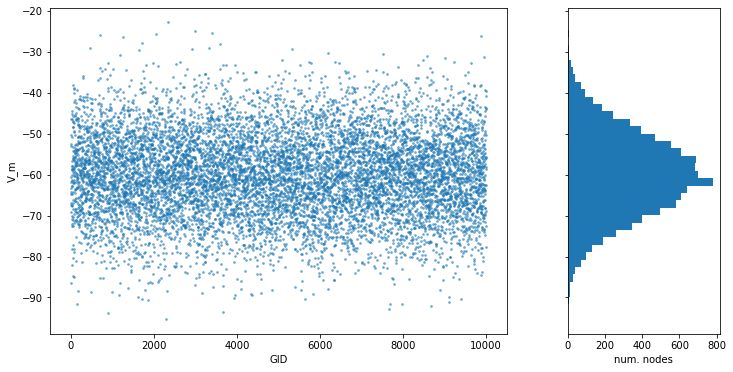## Spatial parameters¶

The spatial module contains parameters related to spatial positions of the nodes.

To create spatially distributed nodes (see section on spatially distributed nodes for more), use nest.spatial.grid() or nest.spatial.free.

Parameter

Description

nest.spatial.grid(shape, center=None,
extent=None, edge_wrap=False)


Create spatially positioned nodes distributed on a grid with dimensions given by shape=[nx, ny(, nz)].

nest.spatial.free(pos, extent=None,
edge_wrap=False, num_dimensions=None)


Create spatially positioned nodes distributed freely in space with dimensions given by pos or num_dimensions.

grid_nodes = nest.Create('iaf_psc_alpha', positions=nest.spatial.grid(shape=[10, 8]))
nest.PlotLayer(grid_nodes);free_nodes = nest.Create('iaf_psc_alpha', 100,
positions=nest.spatial.free(nest.random.uniform(min=0., max=10.),
num_dimensions=2))
nest.PlotLayer(free_nodes);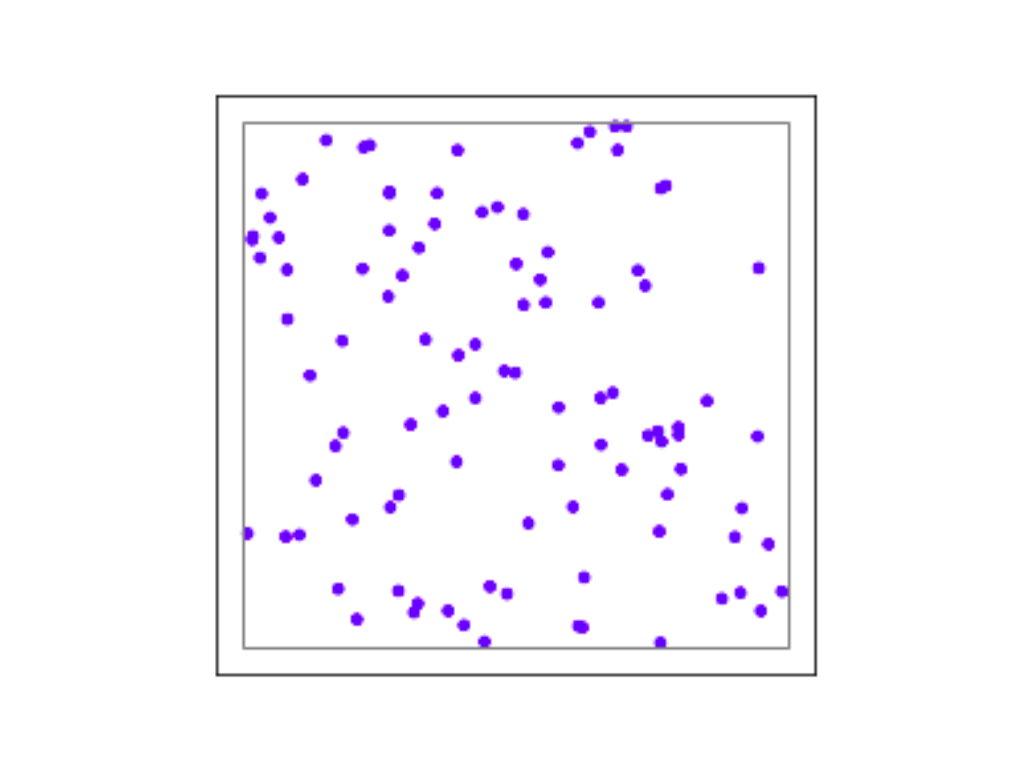After you have created your spatially distributed nodes, you can use the spatial property to set node or connection parameters.

Parameter

Description

nest.spatial.pos.x
nest.spatial.pos.y
nest.spatial.pos.z

Position of a neuron, on the x, y, and z axis.
Can be used to set node properties, but not for connecting.
nest.spatial.source_pos.x
nest.spatial.source_pos.y
nest.spatial.source_pos.z

Position of the source neuron, on the x, y, and z axis.
Can only be used when connecting.
nest.spatial.target_pos.x
nest.spatial.target_pos.y
nest.spatial.target_pos.z

Position of the target neuron, on the x, y, and z axis.
Can only be used when connecting.
nest.spatial.distance

Distance between two nodes. Can only be used when connecting.
nest.spatial.distance.x
nest.spatial.distance.y
nest.spatial.distance.z

Distance on the x, y and z axis between the source and target neuron.
Can only be used when connecting.

These parameters represent positions of neurons or distances between two neurons. To set node parameters, only the node position can be used. The others can be used when connecting.

positions = nest.spatial.free([[x, 0.5*x] for x in np.linspace(0, 1.0, 10000)])
spatial_nodes = nest.Create('iaf_psc_alpha', positions=positions)

parameter = -60 + nest.spatial.pos.x + (0.4 * nest.spatial.pos.x * nest.random.normal())
spatial_nodes.set('V_m'=parameter)

node_pos = np.array(nest.GetPosition(spatial_nodes))
node_pos[:,1]
v_m = spatial_nodes.get('V_m');

fig, ax = pyplot.subplots(figsize=(12, 6))
ax.plot(node_pos[:,0], v_m, '.', ms=3.5)
ax.set_xlabel('Node position on x-axis')
ax.set_ylabel('V_m');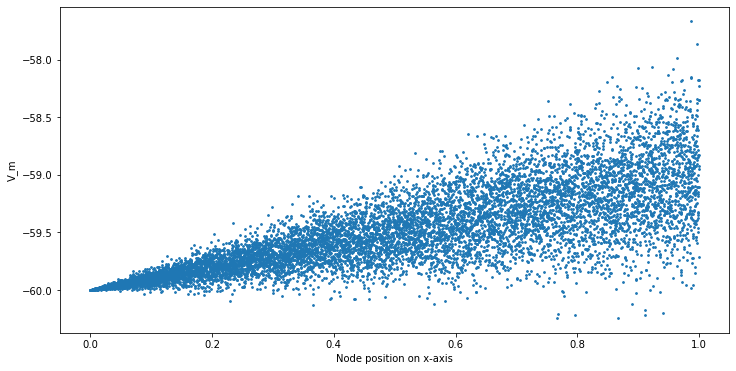## Spatial distribution functions¶

The spatial_distributions module contains random distributions that take a spatial parameter as input and applies the distribution on the parameter. They are used for spatially distributed nodes.

Distribution function

Arguments

Function

nest.spatial_distributions.exponential()

x,
beta
$p(x) = e^{-\frac{x}{\beta}}$

nest.spatial_distributions.gaussian()

x,
mean,
std
$p(x) = e^{-\frac{(x-\text{mean})^2} {2\text{std}^2}}$

nest.spatial_distributions.gaussian2D()

x,
y,
mean_x,
mean_y,
std_x,
std_y,
rho
$p(x) = e^{-\frac{\frac{(x-\text{mean_x})^2} {\text{std_x}^2}+\frac{ (y-\text{mean_y})^2}{\text{std_y}^2}+2 \rho\frac{(x-\text{mean_x})(y-\text{mean_y})} {\text{std_x}\text{std_y}}} {2(1-\rho^2)}}$

nest.spatial_distributions.gamma()

x,
kappa
$p(x) = \frac{x^{\kappa-1}e^{-\frac{x} {\theta}}}{\theta^\kappa\Gamma(\kappa)}$

With these functions, you can recreate for example a Gaussian kernel as a parameter:

 NEST 2.x NEST 3.0 kernel = {"gaussian": {"p_center": 1.0, "sigma": 1.0}}  param = nest.spatial_distributions.gaussian( nest.spatial.distance, p_center=1.0, std_deviation=1.0) 
N = 21
middle_node = N//2

positions = nest.spatial.free([[x, 0.] for x in np.linspace(0, 1.0, N)])
spatial_nodes = nest.Create('iaf_psc_alpha', positions=positions)

parameter = nest.spatial_distributions.exponential(nest.spatial.distance, beta=0.15)

# Iterate connection to get statistical connection data
for _ in range(2000):
nest.Connect(spatial_nodes[middle_node], spatial_nodes,
conn_spec={'rule': 'pairwise_bernoulli',
'p': parameter})

targets = nest.GetConnections().get('target')

fig, ax = pyplot.subplots(figsize=(12, 6))
bars = ax.hist(targets, bins=N, edgecolor='black', linewidth=1.2)

pyplot.xticks(bars + 0.5,np.arange(1, N+1))
ax.set_title('Connections from node with NodeID {}'.format(spatial_nodes[middle_node].get('global_id')))
ax.set_xlabel('Target NodeID')
ax.set_ylabel('Num. connections');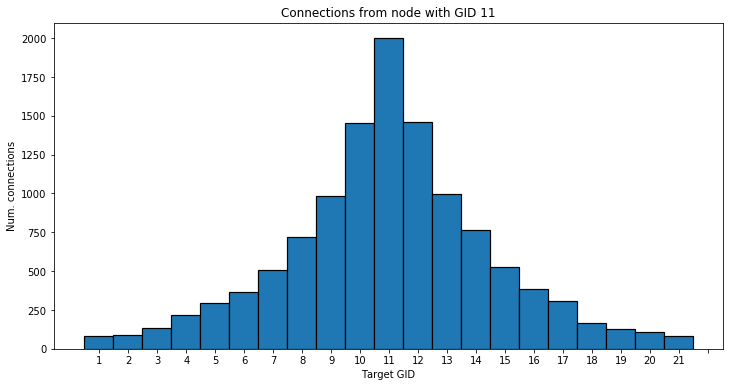## Mathematical functions¶

Parameter

Description

nest.random.exp(x)

Calculates the exponential of a parameter
nest.random.cos(x)

Calculates the cosine of a parameter
nest.random.sin(x)

Calculates the sine of a parameter

The mathematical functions take a parameter object as argument, and return a new parameter which applies the mathematical function on the parameter given as argument.

positions = nest.spatial.free([[x, 0.5*x] for x in np.linspace(0, 1.0, 100)])
spatial_nodes = nest.Create('iaf_psc_alpha', positions=positions)

parameter = -60 + nest.math.exp(nest.spatial.pos.x**4)
# Also available:
#   - nest.math.sin()
#   - nest.math.cos()

spatial_nodes.set({'V_m': parameter})

node_pos = np.array(nest.GetPosition(spatial_nodes))
node_pos[:,1]
v_m = spatial_nodes.get('V_m');

fig, ax = pyplot.subplots(figsize=(12, 6))
ax.plot(node_pos[:,0], v_m, '.', ms=6.5)
ax.set_xlabel('Node position on x-axis')
ax.set_ylabel('V_m');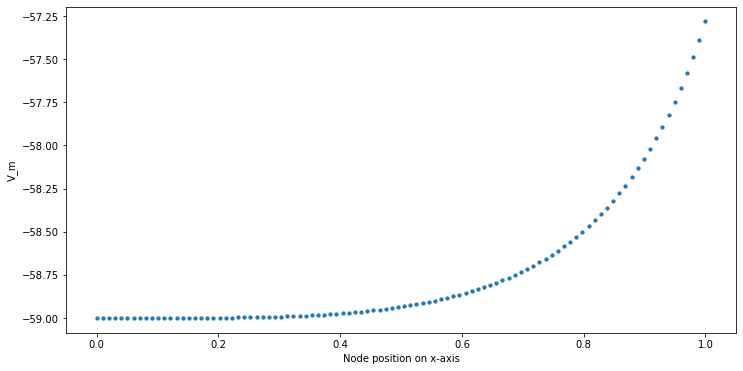## Clipping, redraw, and conditionals¶

Parameter

Description

nest.math.min(x, value)


If a value from the Parameter is above a threshold, x, the value is replaced with the value of the threshold.

nest.math.max(x, value)


If a value from the parameter is below a threshold, x, the value is replaced with the value of the threshold.

nest.math.redraw(x, min, max)


If a value from the parameter is outside of the limits given, the value is redrawn. Throws an error if a suitable value is not found after a certain number of redraws.

nest.logic.conditional(x, val_true, val_false)


Given a condition, yields one value or another based on if the condition evaluates to true or false.

Note that x is a nest.Parameter.

The nest.math.min() and nest.math.max() functions are used to clip a parameter. Essentially they work like the standard min() and max() functions, nest.math.min() yielding the smaller of two values, and nest.math.max() yielding the larger of two values.

# This yields values between 0.0 and 0.5, where values from the
# distribution that are above 0.5 get set to 0.5.
nest.math.min(nest.random.uniform(), 0.5)

# This yields values between 0.5 and 1.0, where values from the
# distribution that are below 0.5 get set to 0.5.
nest.math.max(nest.random.uniform(), 0.5)

# This yields values between 0.2 and 0.7, where values from the
# distribution that are smaller than 0.2 or larger than 0.7 get
# redrawn from the distribution.
nest.math.redraw(nest.random.uniform(), min=0.2, max=0.7)


The nest.logic.conditional() function works like an if/else statement. Three arguments are required:

• The first argument is a condition.

• The second argument is the resulting value or parameter evaluated if the condition evaluates to true.

• The third argument is the resulting value or parameter evaluated if the condition evaluates to false.

# A Heaviside step function with uniformly distributed input values.
nest.logic.conditional(nest.random.uniform(min=-1., max=1.) < 0., 0., 1.)

positions = nest.spatial.free([[x, 0.5*x] for x in np.linspace(0, 1.0, 50)])
spatial_nodes = nest.Create('iaf_psc_alpha', positions=positions)

spatial_nodes.set(V_m=nest.logic.conditional(nest.spatial.pos.x < 0.5,
-55 + 10*nest.spatial.pos.x,
-55))

node_pos = np.array(nest.GetPosition(spatial_nodes))
node_pos[:,1]
v_m = spatial_nodes.get('V_m');

fig, ax = pyplot.subplots(figsize=(12, 6))
ax.plot(node_pos[:,0], v_m, 'o')
ax.set_xlabel('Node position on x-axis')
ax.set_ylabel('V_m');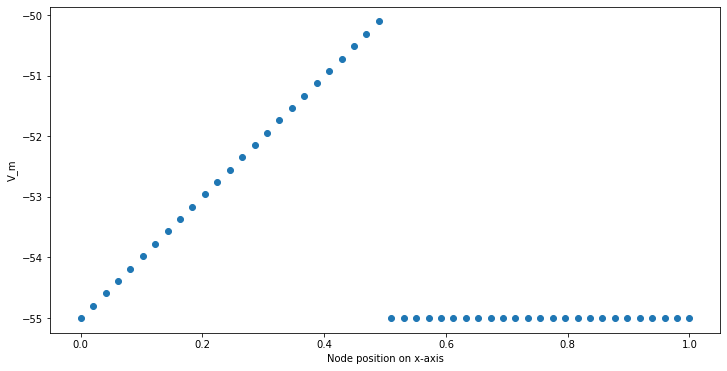## Compare parameters¶

In the nest.logic.conditional() function above, we compared a nest.Parameter with a value. It is also possible to compare one nest.Parameter with another. All comparison operators are supported. The result of such comparisons is a new nest.Parameter, which evaluates to either 1 or 0 for true and false, respectively. The resulting comparison nest.Parameter can be used in a nest.logic.conditional(). You can omit the nest.logic.conditional() if the desired result is zero when the comparison is false.

# As an example, take a step function where the resulting value is
# 0.5 for positive values and 0 for negative values.
nest.logic.conditional(nest.random.uniform(min=-1., max=1.) > 0.0, 0.5, 0.0)

# This comparison can be used without the nest.logic.conditional() function.
0.5*(nest.random.uniform(min=-1., max=1.) > 0.0)


## Combine parameters¶

NEST parameters support the basic arithmetic operations. Two parameters can be added together, subtracted, multiplied with each other, or one can be divided by the other. They also support being raised to the power of a number, but they can only be raised to the power of an integer or a floating point number. Parameters can therefore be combined in almost any way. In fact the distribution functions in nest.spatial_distributions are just arithmetic expressions defined in Python.

Some examples:

# A uniform distribution yielding values in the range (-44., -64.).
p = -54. + nest.random.uniform(min=-10., max=10)

# Two random distributions combined, with shifted center.
p = 1.0 + 2 * nest.random.exponential() * nest.random.normal()

# The node position on the x-axis, combined with a noisy y-axis component.
p = nest.spatial.pos.x + (0.4 * nest.spatial.pos.y * nest.random.normal())

# The quadratic distance between two nodes, with a noisy distance component.
p = nest.spatial.distance**2 + 0.4 * nest.random.uniform() * nest.spatial.distance


## Use parameters to set node properties¶

Using parameters makes it easy to set node properties

NEST 2.x

NEST 3.0

for gid in nrns:
v_m = numpy.random.uniform(-20., 20.)
nest.SetStatus([node_id], {'V_m': V_m})

nrns.V_m=nest.random.uniform(-20., 20)